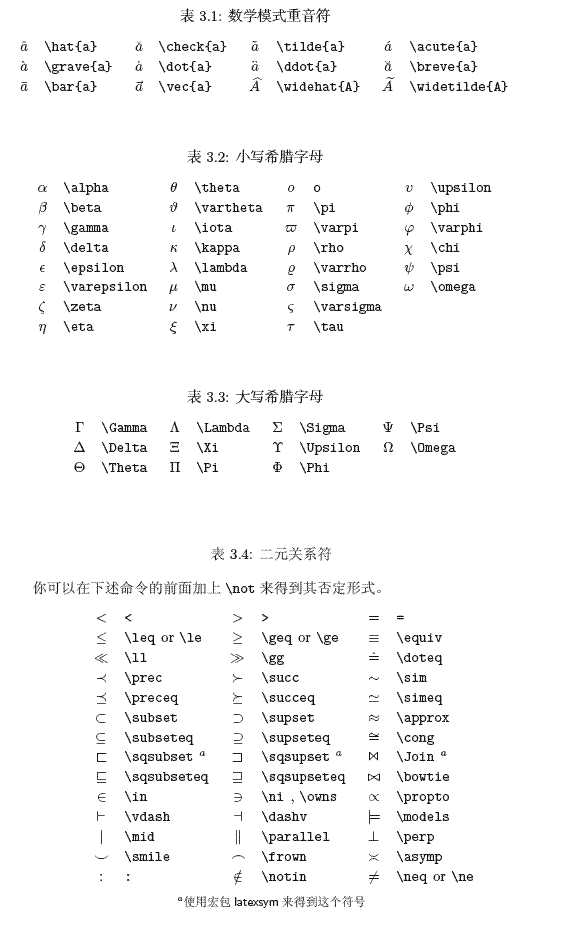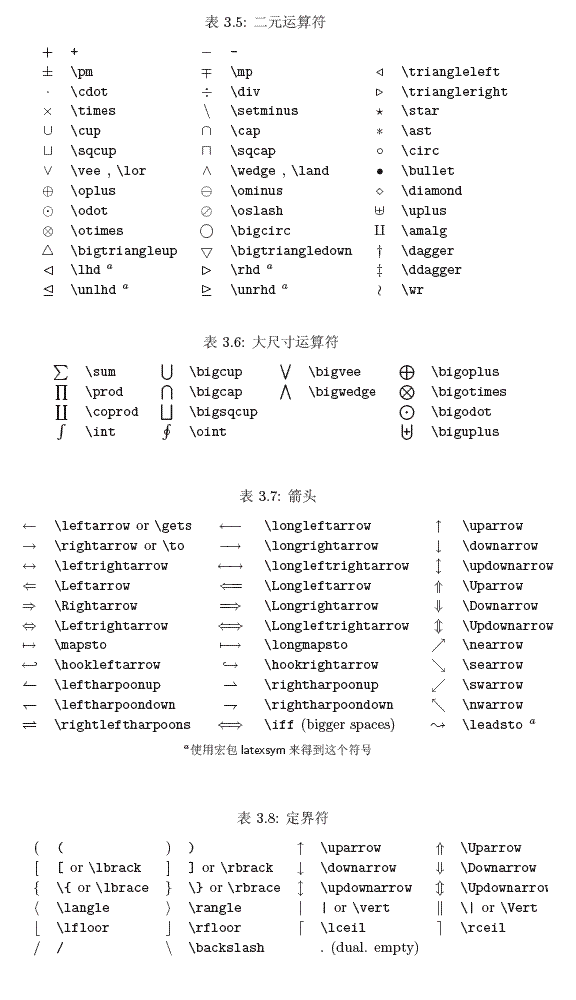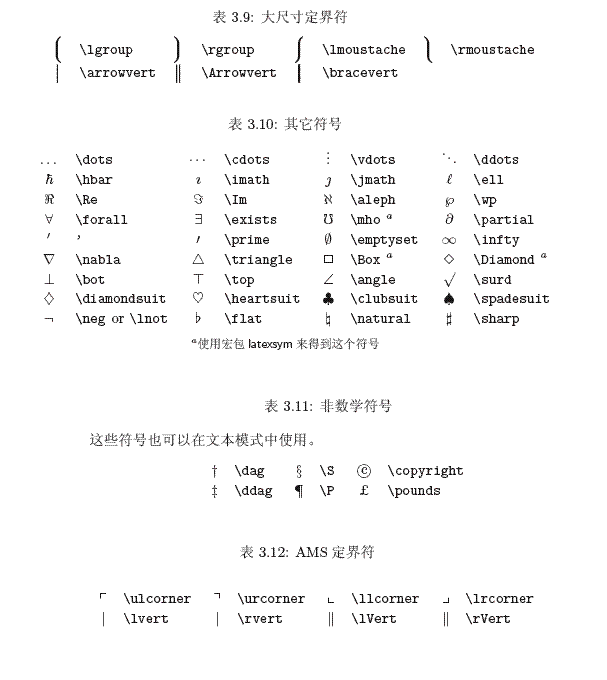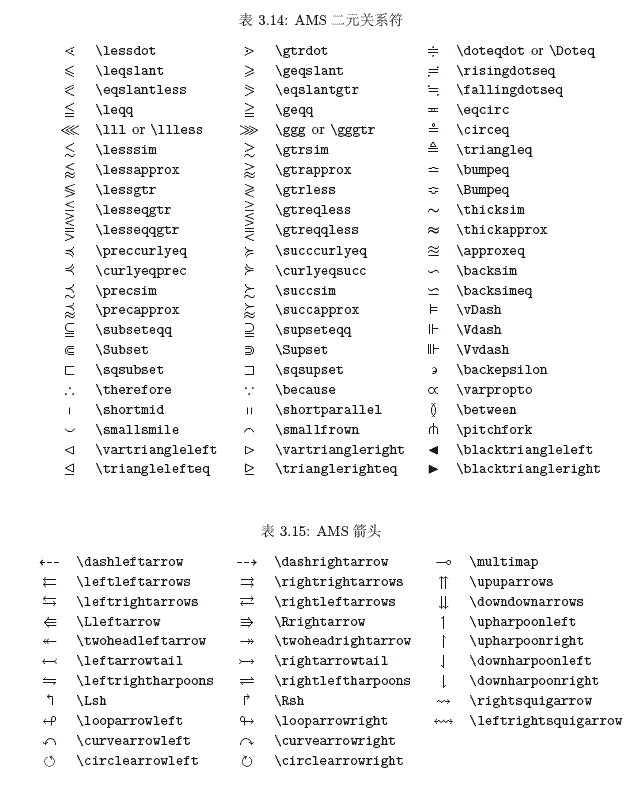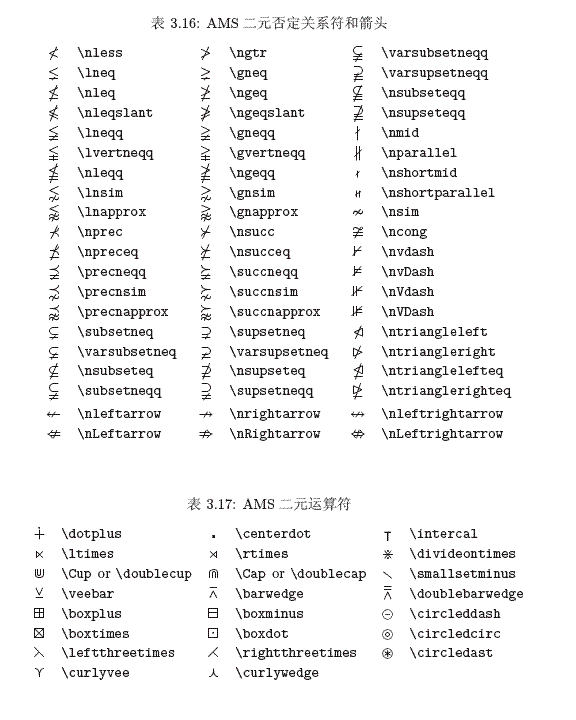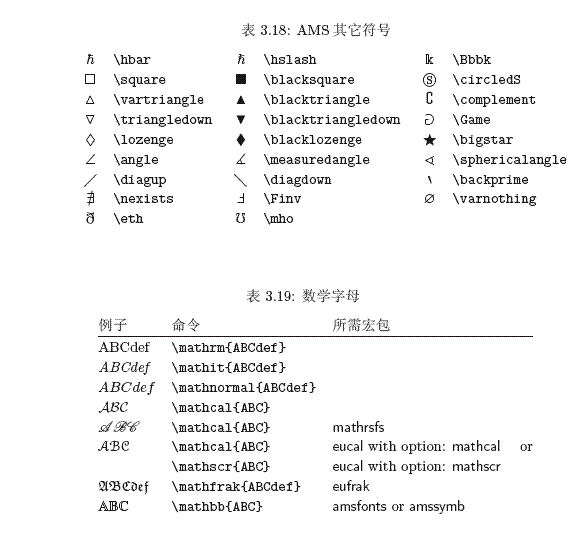# 我的LaTeX秘籍（不断更新中）

## 关于算法的排版

\par Input: Data set $D$
\\
\hangafter 1 \hangindent 5.1em \noindent
Distance function $f$ (e.g., Euclidean distance)
\par //Initialisation: the whole data space needs to be insepected
\\
\hangafter 1 \hangindent 3.5em \noindent
$\mathcal{T}={(\infty,\infty)}$
\par //Loop: iterate until all regions have been investigated
\\
\hangafter 1 \hangindent 3.5em \noindent
WHILE ($\mathcal{T}\not=\emptyset$) DO\\
\hangindent 3.5em \indent
$(m_x,m_y)$=takeElement($\mathcal{T}$)\\
\hangindent 3.5em \indent
IF($\exists$ boundedNNSearch($O, D, (m_x,m_y), f$)) THEN\\
\setlength{\parindent}{1.8cm}
\hangindent 3.5em \indent
$(n_x, n_y) =$boundedNNSearch($O, D, (m_x,m_y), f$))\\
\hangindent 3.5em \indent
$\mathcal{T}=\mathcal{T}\cup{(n_x, n_y),(m_x, m_y)}$\\
\hangindent 3.5em \indent
OUTPUT $n$\\
\setlength{\parindent}{1cm}
\hangindent 3.5em \indent
END IF\\
\hangafter 1 \hangindent 3.5em \noindent
END WHILE\\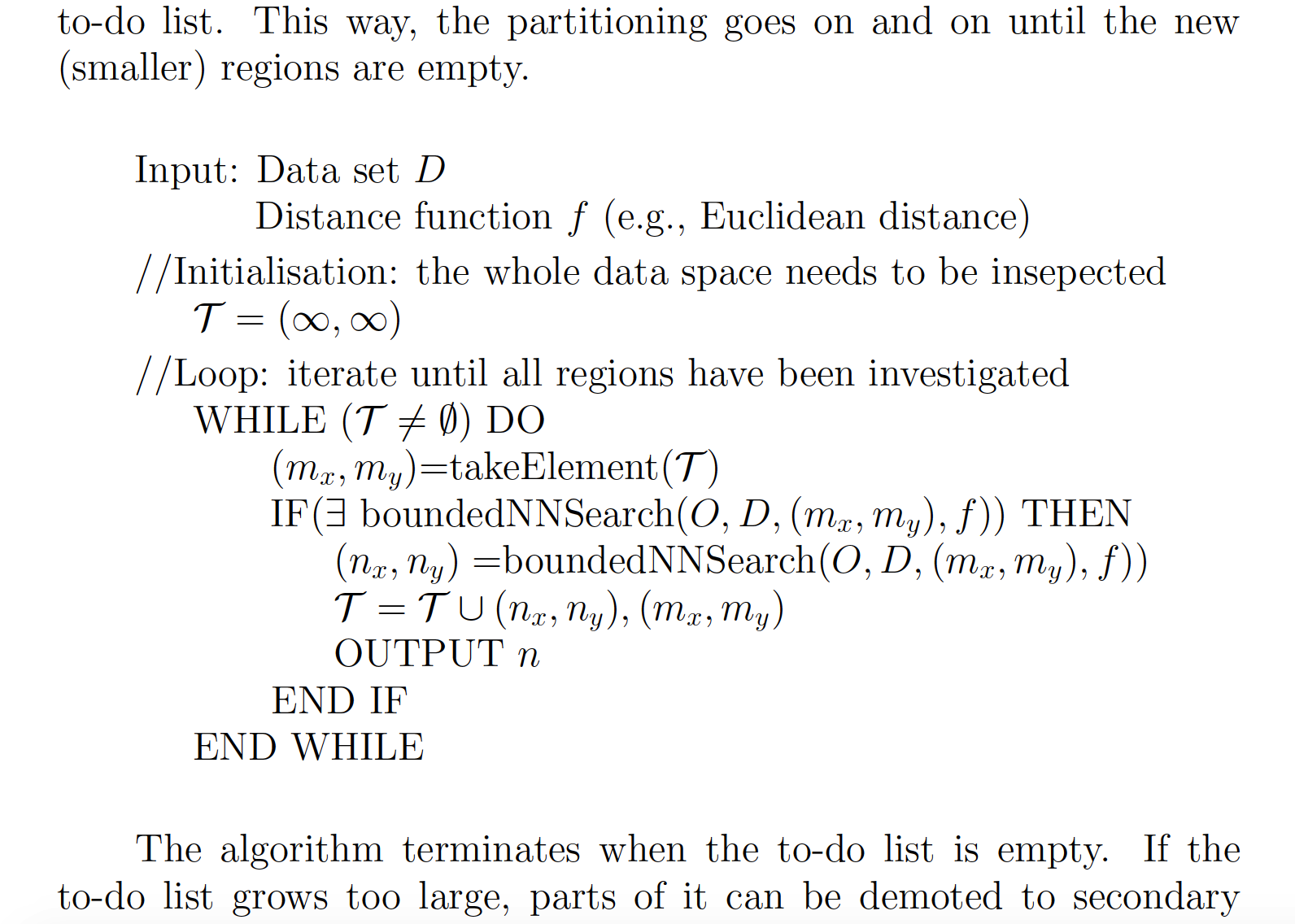\usepackage[linesnumbered,boxed]{algorithm2e}

\begin{algorithm} \SetAlgoNoLine
\caption{The \textit{GeoGreedy} Algorithm}
\KwIn{A data set of $d$-dimensional points $D$ and a desired output size $k$.}
\KwOut{A subset of $D$ of size $k$, denoted by $S$.}
$S\leftarrow \emptyset$\\
\For {each $i:=1, 2, \cdots , d$,}
{
$p \leftarrow$ an $i$-th dimension boundary point of $D$\\
$S \leftarrow S\cup \{p\}$\
}
\For{each $i:=1, 2, \cdots , k-d$,}
{
find a point $p \in D \setminus S$ with the smallest critical ratio for $S$ (i.e., $cr(p,S)$)\\
\If{$cr(p,S)\geq 1$}
{
\textbf{return} $S$\\
}
\Else
{
$S \leftarrow S\cup \{p\}$\\
}
}
\textbf{return} $S$\
\end{algorithm}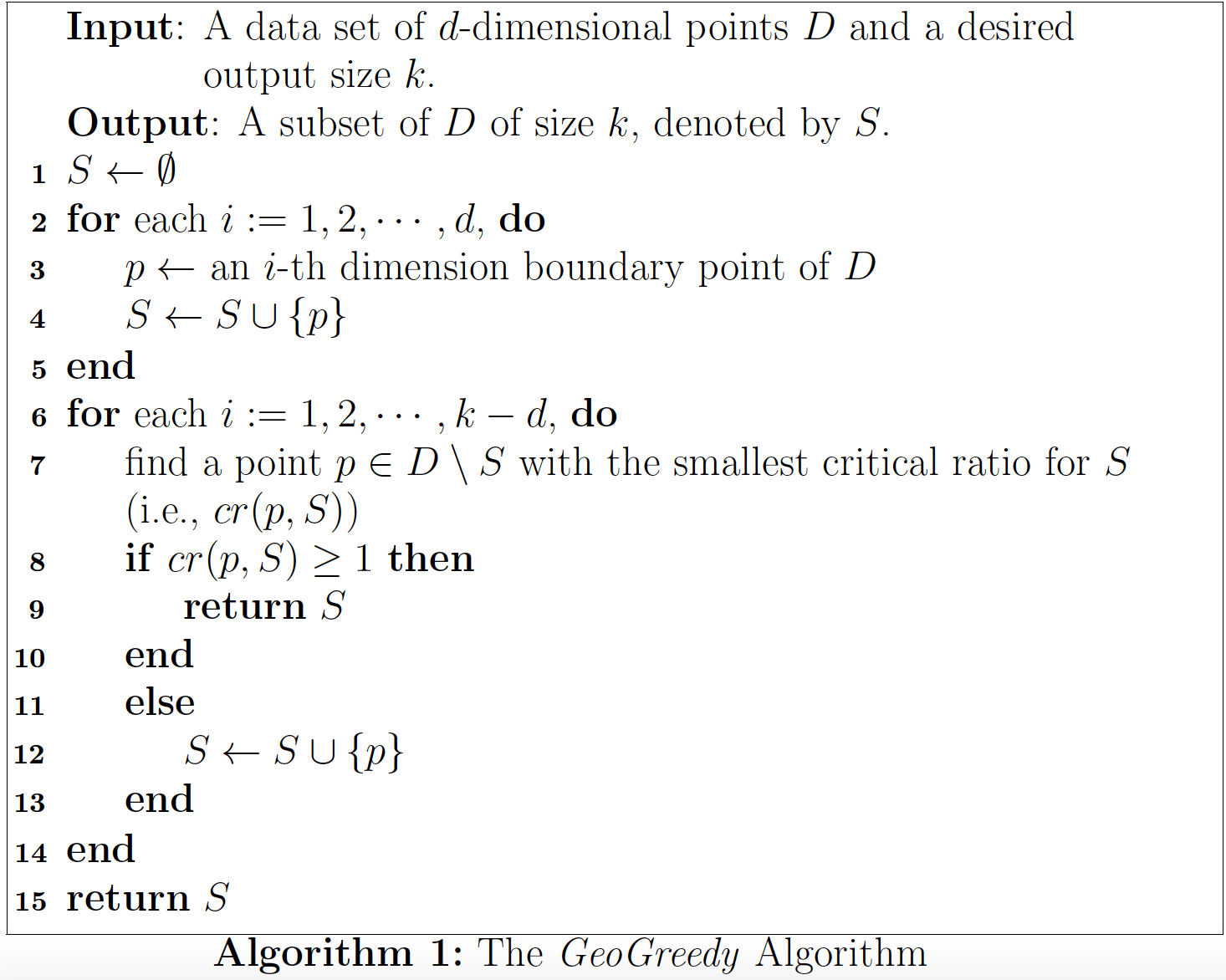## 控制列表项的行距与段间隔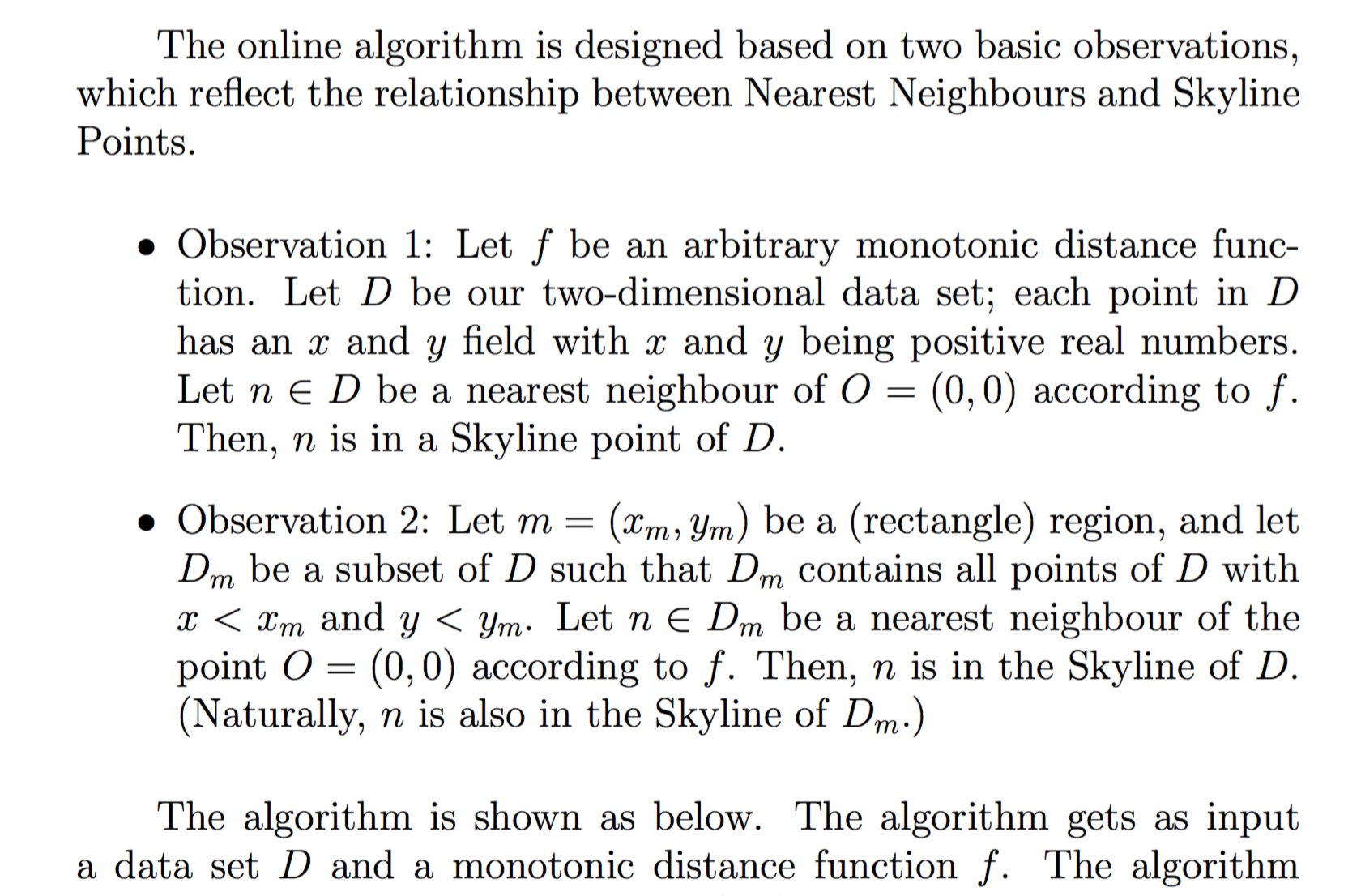In this paper, there types of utility functions are considered:
\begin{itemize}[leftmargin=3.2em]
\vspace{-0.8em}
\setlength{\itemsep}{0pt}
\setlength{\parsep}{0pt}
\setlength{\parskip}{0pt}
\item Convex Function
\item Concave Function
\item Constant Elasticity of Substitution (CES) Function.
\vspace{-0.8em}
\end{itemize}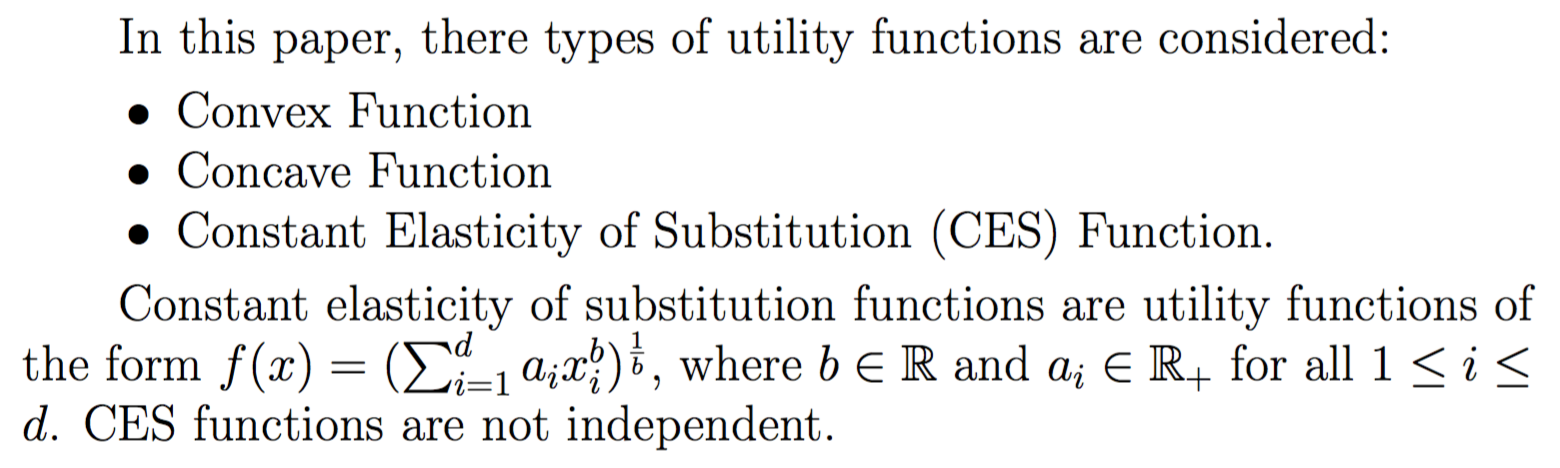## 自定义方法输入argmax型公式

\usepackage{amsmath}
\usepackage{amssymb}
\DeclareMathOperator*{\argmax}{argmax}

$$p_i^* = \mathop{\argmax}_{p\in{D}}{(p[i])}$$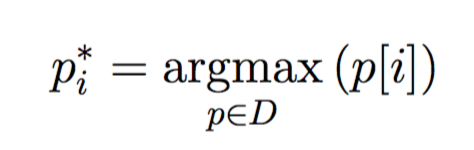## 多行公式的对齐

Also, we can conclude the the following inequalities
\begin{align*}
\sum\limits_{i=1}^{d-1}(p[i]-s[i])\cdot v[i] &\leq \sum\limits_{i=1}^{d-1}\frac{c_i}{t}v[i]\\
&\leq \frac{d-1}{t} \max_{i\leq d-1}{(c_iv[i])}\\
&\leq \frac{d-1}{t} \max_{i\leq d-1}{(p_i^* \cdot v)}
\end{align*}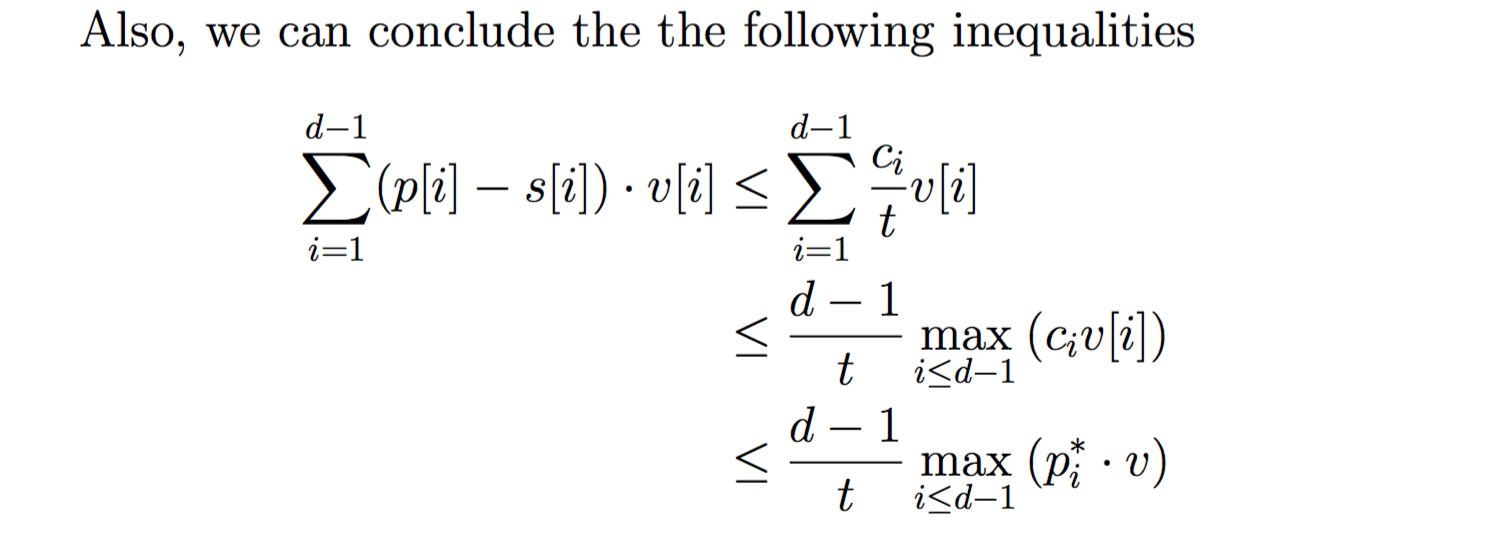## 关于参考文献

\begin{thebibliography}{1}
\bibitem{}
Donald Kossmann, Frank Ramsak, Steffen Rost, 2002,
\emph{Shooting Stars in the Sky: An Online Algorithm for Skyline Queries},
Proceedings of the 28th VLDB Conference, Hong Kong, China.
\end{thebibliography}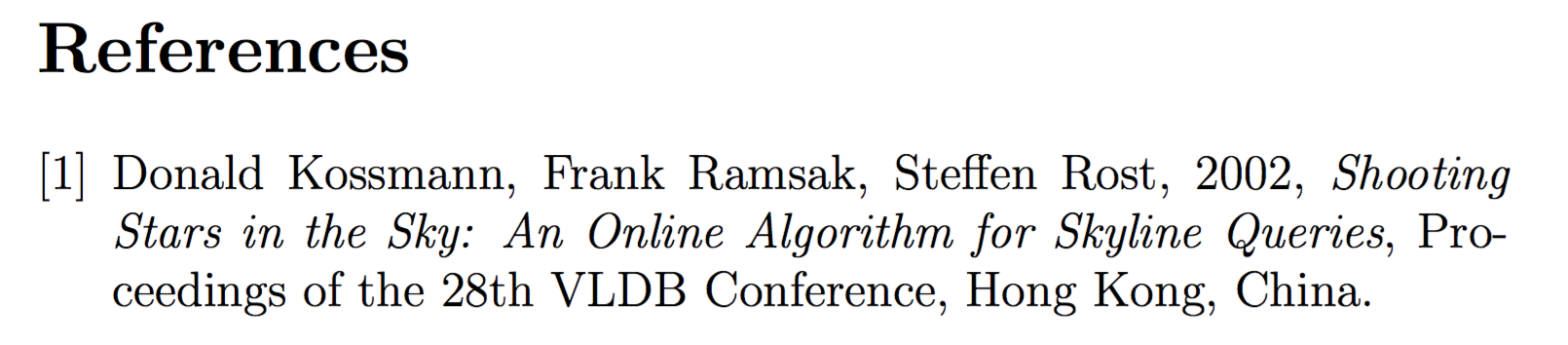\section{Overview}
Geometry approach for $k$-regret query is proposed in~\cite{peng2014geometry}.
This new algorithm takes advantage of some geometry property to improve
the performance of \textsc{Cube}~\cite{nanongkai2010regret}.

\bibliographystyle{unsrt}%
\bibliography{Ref_GeoApp}

@inproceedings{peng2014geometry,
title={Geometry approach for k-regret query},
author={Peng, Peng and Wong, Raymond Chi-Wing},
booktitle={ICDE},
pages={772--783},
year={2014}
}

@article{nanongkai2010regret,
title={Regret-minimizing representative databases},
author={Nanongkai, Danupon and Sarma, Atish Das and Lall, Ashwin and Lipton, Richard J and Xu, Jun},
journal={Proceedings of the VLDB Endowment},
volume={3},
number={1-2},
pages={1114--1124},
year={2010}
}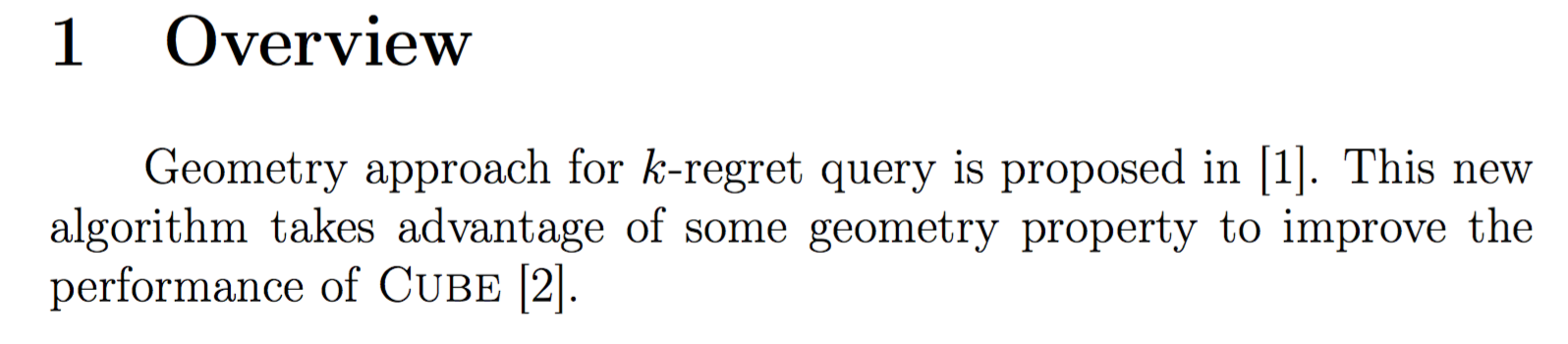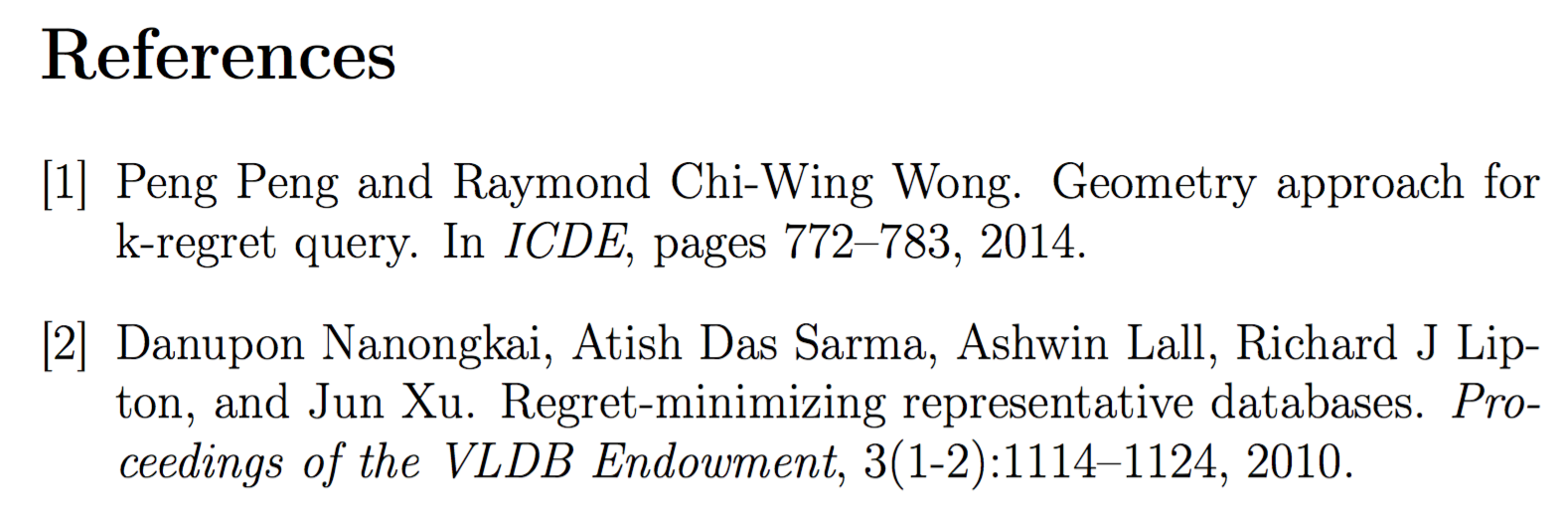## 输入类似分段函数的大括号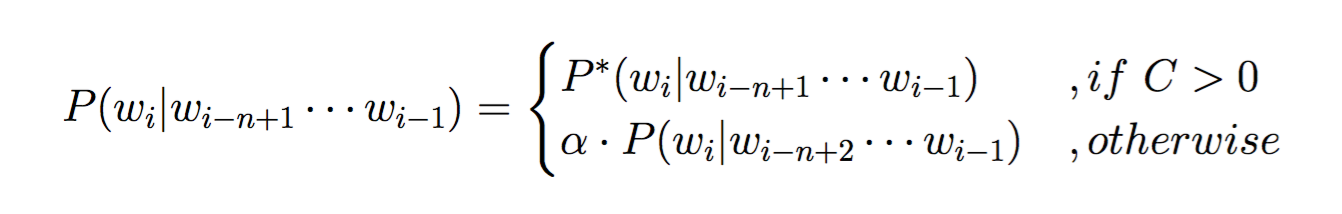$$P(w_i|w_{i-n+1}\cdots w_{i-1})=\begin{cases} P^*(w_i|w_{i-n+1}\cdots w_{i-1})&,if\ C>0\\ \alpha \cdot P(w_i|w_{i-n+2}\cdots w_{i-1})&,otherwise \end{cases}$$

## 关于首行缩进的问题

\documentclass[a4paper]{article}
\usepackage[utf8]{inputenc}

\title{A Study In Scarlet}
\author{Arthur Conan Doyle}
\date{}

\begin{document}
\maketitle

In the year 1878 I took my degree % 省略后续文字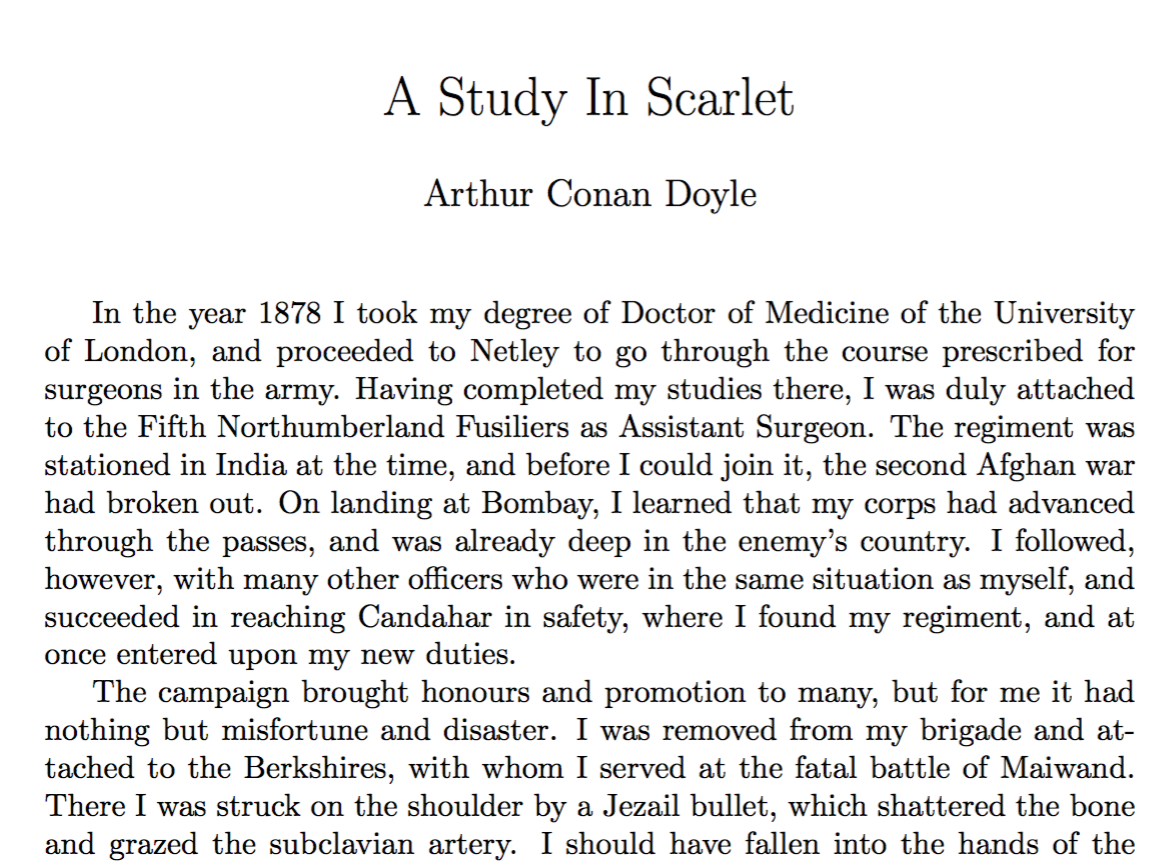\documentclass[a4paper, 14pt]{extarticle}
\usepackage[utf8]{inputenc}

\title{\huge{\textsc{A Study In Scarlet}}}
\author{Arthur Conan Doyle}
\date{}

\begin{document}
\maketitle

\section*{Chapter 1 - Mr. Sherlock Holmes}
In the year 1878 I took my degree % 省略后续文字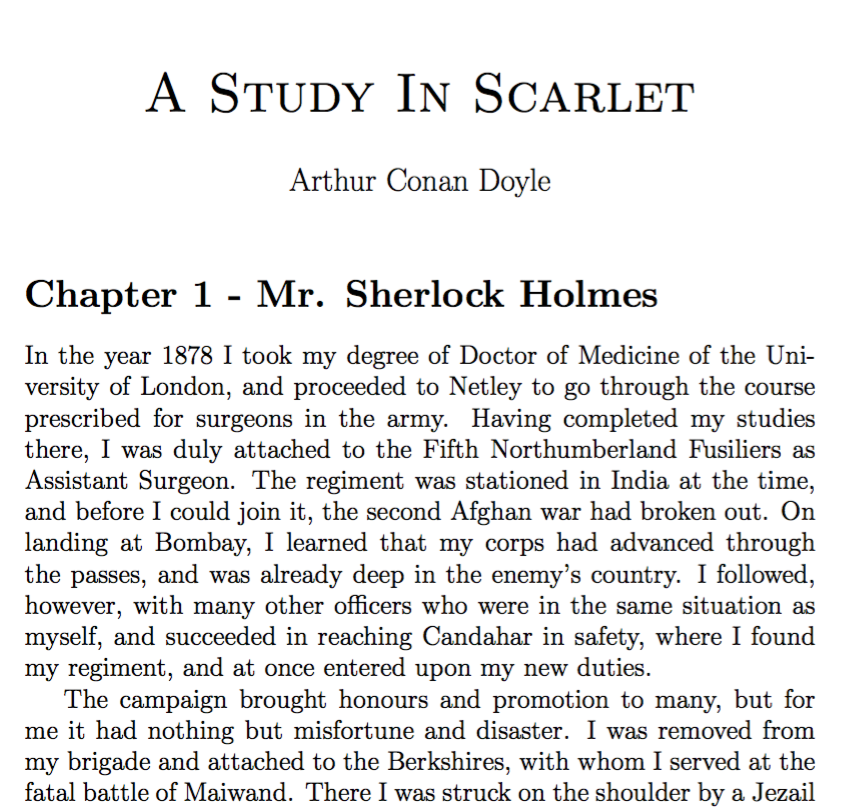\documentclass[a4paper, 14pt]{extarticle}
\usepackage[utf8]{inputenc}
\usepackage{indentfirst}
\setlength{\parindent}{2em}
\addtolength{\parskip}{3pt}

\title{\huge{\textsc{A Study In Scarlet}}}
\author{Arthur Conan Doyle}
\date{}

\begin{document}
\maketitle

\section*{Chapter 1 - Mr. Sherlock Holmes}
In the year 1878 I took my degree % 省略后续文字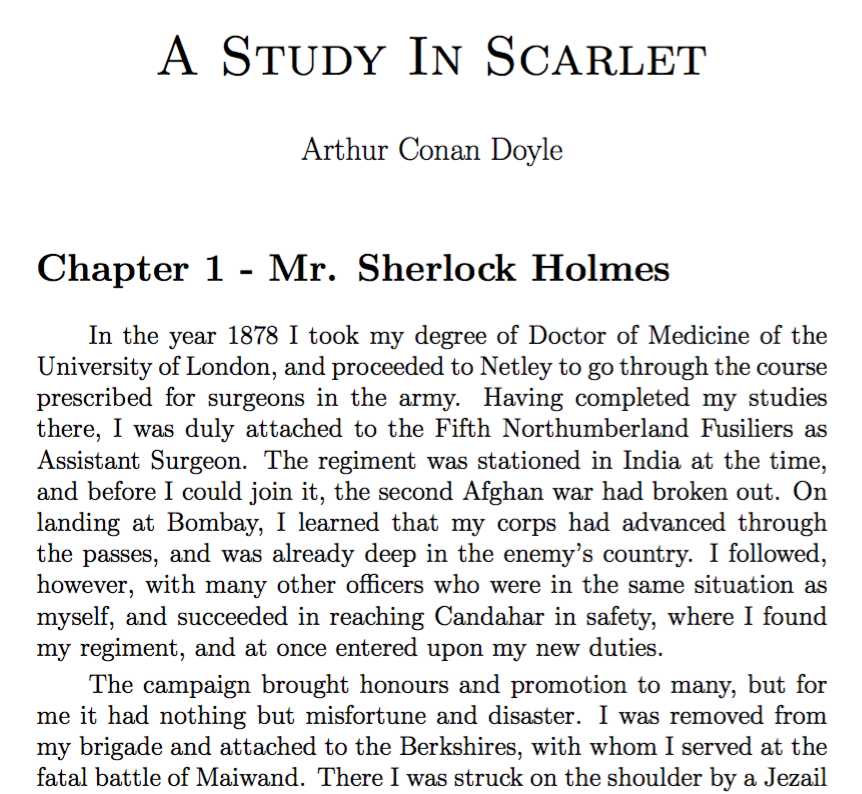## 如何输入矩阵

$$\mathbf{H}= \begin{bmatrix} \frac{\partial^2f}{\partial x_1^2} & \frac{\partial^2f}{\partial x_1\partial x_2} & \cdots &\frac{\partial^2f}{\partial x_1\partial x_n} \\ \frac{\partial^2f}{\partial x_2\partial x_1} & \frac{\partial^2f}{\partial x_2^2} & \cdots &\frac{\partial^2f}{\partial x_2\partial x_n} \\ \vdots & \vdots & \ddots & \vdots \\ \frac{\partial^2f}{\partial x_n\partial x_1}&\frac{\partial^2f}{\partial x_n\partial x_2}& \cdots &\frac{\partial^2f}{\partial x_n^2} \end{bmatrix}$$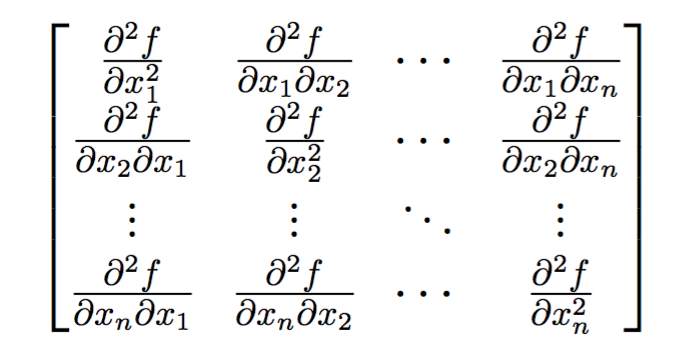## 行内公式显示模式的调整技巧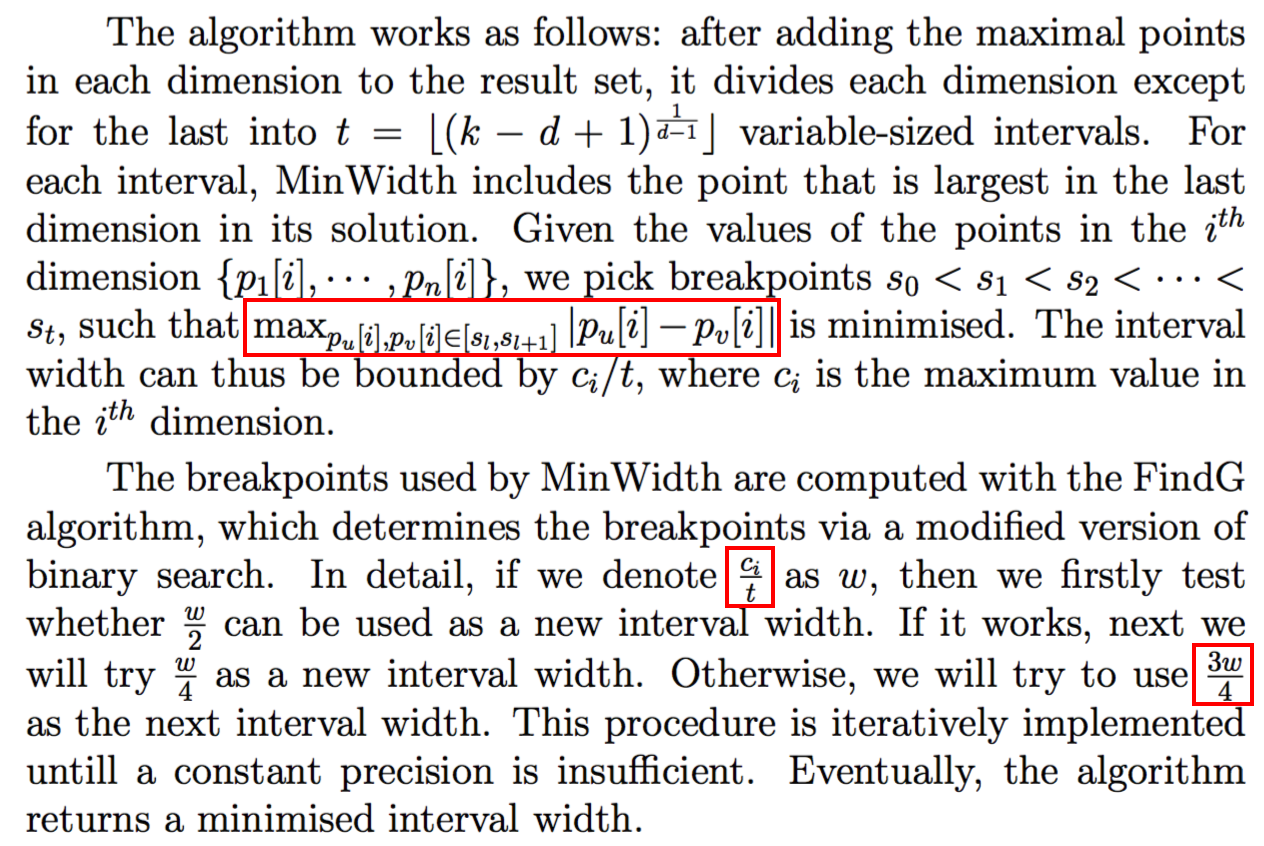$\displaystyle \max_{p_u[i],p_v[i]\in[s_l,s_{l+1}]}|p_u[i]-p_v[i]|$
\$\displaystyle \frac{c_i}{t}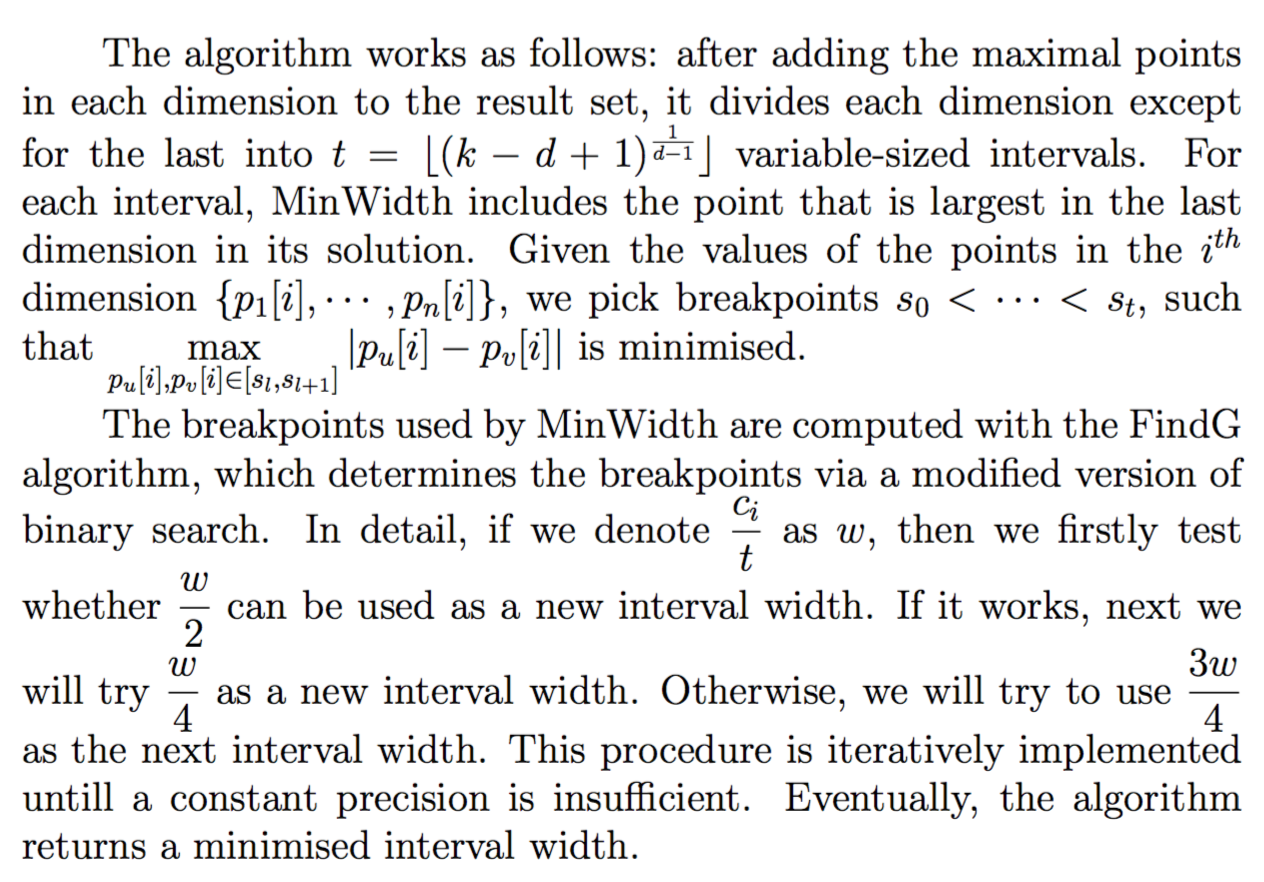## 附录——常用数学符号表